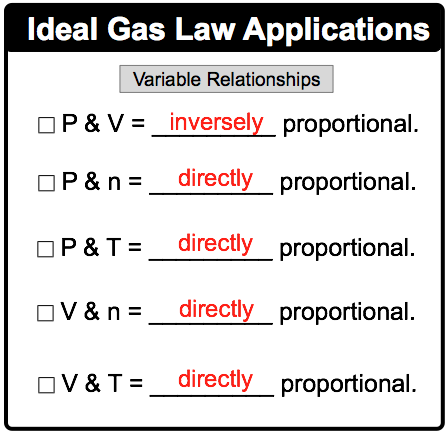Clutch Prep is now a part of Pearson

# The Ideal Gas Law Applications

See all sections
Sections
Pressure Units
The Ideal Gas Law
The Ideal Gas Law Derivations
The Ideal Gas Law Applications
Chemistry Gas Laws
Chemistry Gas Laws: Combined Gas Law
Mole Fraction
Partial Pressure
The Ideal Gas Law: Molar Mass
The Ideal Gas Law: Density
Gas Stoichiometry
Standard Temperature and Pressure
Effusion
Root Mean Square Speed
Kinetic Energy of Gases
Maxwell-Boltzmann Distribution
Velocity Distributions
Kinetic Molecular Theory
Van der Waals Equation
Boyle's Law (IGNORE)
Charles Law (IGNORE)
Ideal Gas Law (IGNORE)

The Ideal Gas Law Applications establish both the direct and inverse relationships between the Ideal Gas Law variables.

###### Understanding the Ideal Gas Law Applications

Concept #1: The Ideal Gas Law ApplicationsExample #1: If the number of moles (n) inside a container were tripled while keeping the pressure (P) constant, whatwill happen to the volume (V)?

Practice: The relationship between the partial pressure of a gas (P) and the number of moles of that gas (n) is best represented by which of the following graphs?